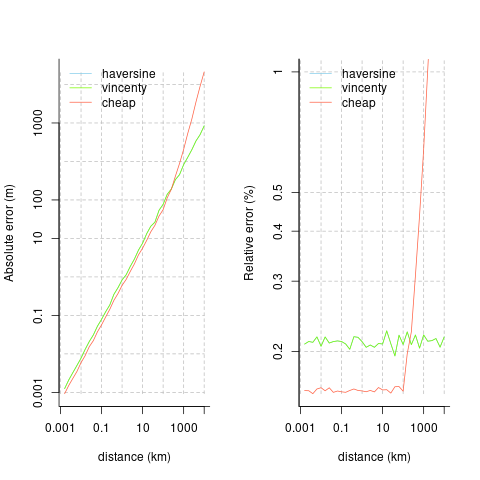# geodist

An ultra-lightweight, zero-dependency package for very fast calculation of geodesic distances. Main eponymous function, geodist(), accepts only one or two primary arguments, which must be rectagular objects with unambiguously labelled longitude and latitude columns (that is, some variant of lon/lat, or x/y).

n <- 50
x <- cbind (-10 + 20 * runif (n), -10 + 20 * runif (n))
y <- cbind (-10 + 20 * runif (2 * n), -10 + 20 * runif (2 * n))
colnames (x) <- colnames (y) <- c ("x", "y")
d0 <- geodist (x) # A 50-by-50 matrix
d1 <- geodist (x, y) # A 50-by-100 matrix
d2 <- geodist (x, sequential = TRUE) # Vector of length 49
d2 <- geodist (x, sequential = TRUE, pad = TRUE) # Vector of length 50

## Detailed Usage

Input(s) to the geodist() function can be in arbitrary rectangular format.

n <- 1e1
x <- tibble::tibble (x = -180 + 360 * runif (n),
y = -90 + 180 * runif (n))
dim (geodist (x))
#>  10 10
y <- tibble::tibble (x = -180 + 360 * runif (2 * n),
y = -90 + 180 * runif (2 * n))
dim (geodist (x, y))
#>  10 20
x <- cbind (-180 + 360 * runif (n),
-90 + 100 * runif (n),
seq (n), runif (n))
colnames (x) <- c ("lon", "lat", "a", "b")
dim (geodist (x))
#>  10 10

All outputs are distances in metres, calculated with a variety of spherical and elliptical distance measures. Distance measures currently implemented are Haversine, Vincenty (spherical), the very fast mapbox cheap ruler (see their blog post), and the “reference” implementation of Karney (2013), as implemented in the package sf. (Note that geodist does not accept sf-format objects; the sf package itself should be used for that.) Note that The mapbox cheap ruler algorithm is intended to provide approximate yet very fast distance calculations within small areas (typically the size of single cities or study sites).

### Benchmarks of geodesic accuracy

The geodist_benchmark() function - the only other function provided by the geodist package - compares the accuracy of the different metrics to the nanometre-accuracy standard of Karney (2013).

geodist_benchmark (lat = 30, d = 1000)
#>            haversine    vincenty       cheap
#> absolute 0.836551561 0.836551562 0.594188257
#> relative 0.002155514 0.002155514 0.001616718

All distances (d) are in metres, so that result indicates that all measures are accurate to within 1m over distances out to several km. The following plots compare the absolute and relative accuracies of the different distance measures implemented here. The mapbox cheap ruler algorithm is the most accurate for distances out to around 100km, beyond which it becomes extremely inaccurate. Average relative errors of Vincenty distances remain generally constant at around 0.2%, while relative errors of cheap-ruler distances out to 100km are around 0.16%.### Performance comparison

The following code demonstrates the relative speed advantages of the different distance measures implemented in the geodist package.

n <- 1e3
dx <- dy <- 0.01
x <- cbind (-100 + dx * runif (n), 20 + dy * runif (n))
y <- cbind (-100 + dx * runif (2 * n), 20 + dy * runif (2 * n))
colnames (x) <- colnames (y) <- c ("x", "y")
rbenchmark::benchmark (replications = 10, order = "test",
d1 <- geodist (x, measure = "cheap"),
d2 <- geodist (x, measure = "haversine"),
d3 <- geodist (x, measure = "vincenty"),
d4 <- geodist (x, measure = "geodesic")) [, 1:4]
#>                                      test replications elapsed relative
#> 1     d1 <- geodist(x, measure = "cheap")           10   0.058    1.000
#> 2 d2 <- geodist(x, measure = "haversine")           10   0.185    3.190
#> 3  d3 <- geodist(x, measure = "vincenty")           10   0.276    4.759
#> 4  d4 <- geodist(x, measure = "geodesic")           10   3.106   53.552

Geodesic distance calculation is available in the sf package. Comparing computation speeds requires conversion of sets of numeric lon-lat points to sf form with the following code:

require (magrittr)
x_to_sf <- function (x)
{
sapply (seq (nrow (x)), function (i)
sf::st_point (x [i, ]) %>%
sf::st_sfc ()) %>%
sf::st_sfc (crs = 4326)
}
n <- 1e2
x <- cbind (-180 + 360 * runif (n), -90 + 180 * runif (n))
colnames (x) <- c ("x", "y")
xsf <- x_to_sf (x)
sf_dist <- function (x) sf::st_distance (x, x)
geo_dist <- function (x) geodist (x, measure = "geodesic")
rbenchmark::benchmark (replications = 10, order = "test",
sf_dist (xsf),
geo_dist (x)) [, 1:4]
#> Linking to GEOS 3.6.2, GDAL 2.3.0, proj.4 5.0.1
#>           test replications elapsed relative
#> 2  geo_dist(x)           10   0.066    1.000
#> 1 sf_dist(xsf)           10   0.210    3.182

Confirm that the two give almost identical results:

ds <- matrix (as.numeric (sf_dist (xsf)), nrow = length (xsf))
dg <- geodist (x, measure = "geodesic")
formatC (max (abs (ds - dg)), format = "e")
#>  "7.4506e-09"

All results are in metres, so the two differ by only around 10 nanometres.

The geosphere package also offers sequential calculation which is benchmarked with the following code:

fgeodist <- function () geodist (x, measure = "vincenty", sequential = TRUE)
fgeosph <- function () geosphere::distVincentySphere (x)
rbenchmark::benchmark (replications = 10, order = "test",
fgeodist (),
fgeosph ()) [, 1:4]
#>         test replications elapsed relative
#> 1 fgeodist()           10   0.022    1.000
#> 2  fgeosph()           10   0.048    2.182